Budget cash flow and profit and loss amounts to be different for salary & wages

Salary & wages:- want budget cash flow and profit and loss amounts to be different.

The cash flow for salaries and wages is say \$1,000 per month.However, in the profit & loss account you want to show the expense as a function of the number of days worked per month.

A client pays Bill wages of \$1,000 per month which is a total \$12,000 for year.

In the budget cash flow you want:

\$ per month

Jan      1,000

Feb      1,000

Mar      1,000

Total for year will be \$12,000

However, you do not want the P&L or Income statement to show the \$1,000. Instead you want to work out the number of days Bill will work each month and allocate the \$12,000 accordingly. So in the P&L you want:

\$ per month

Jan      1,150

Feb        950

Mar      1,050

Solution

A sample model demonstrated this solution. To get this sample  Right click here and choose Save as (or Save target/link as).

Bring this sample model into Visual Cash Focus by opening any model to get to the map.

• Then choose the File menu (at very top of page) and go to the Export / Import option.
• Then choose Zip or Unzip a model. On the form, initially Save is selected. Instead click on Retrieve, then click the Do it! button.
• Locate the wage_show.zip file that you downloaded above. Click Import. Click OK to the message.
• Give it a modelcode, for example WAGETEST and then click Proceed.

Use the notes below to work through the model.

The total wages amount is \$12,000 for the year. The total number of days worked for the year is 262

The table below shows the number of days worked per month. This is used to allocate the total wages amount of \$12,000 to each month.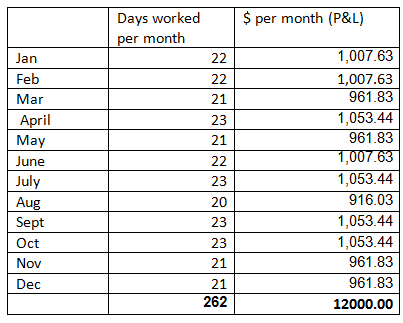To enter this into Visual Cash Focus:

1. In Accounts and budgets, create a new Miscellaneous item called: Days worked per month
2. On Budget per period page: Fill in the number of Days worked for each month: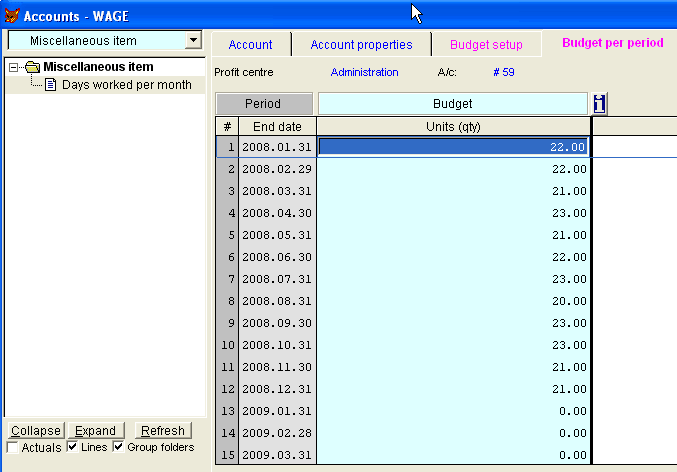Budget setup

Go to the Expense accounts, to Wages. Then click on the Budget setup page: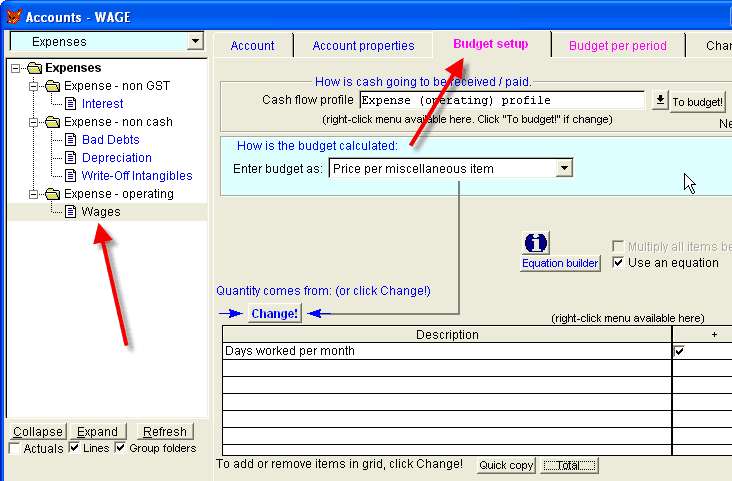For Enter budget as choose: Price per miscellaneous item. Click on Change and choose the Days worked per month miscellaneous item.

The amount for each month is calculated in the table above (under the Solution heading) as:

Amount = days worked in month
——————– x Total wages for year
Total days worked in year

For the first month, it is:
Amount = 22
—– x 12,000 = \$1,007.63
262

Use the Equation functionality of the software to do this calculation, as follows:-

1. Check Use an equation (on the same page).
2. Click on the Equation builder button.

Enter the Equation as appropriate
e.g. {100}/262*12000
The \$12,000 is the total wages amount paid for the year. The 262 is the total number of paid days in a year.

Note: The {100} is an Account

To see the Equation in words, click on Show account description.  You see the following:
—————————
{Days worked per month}/262*12000
Note: The result of this equation will be multiplied by the AMOUNTS on the budget per period page for Wages.
—————————
The info button next to the Show account description button has additional information.
Wages – go to the Budget per period page.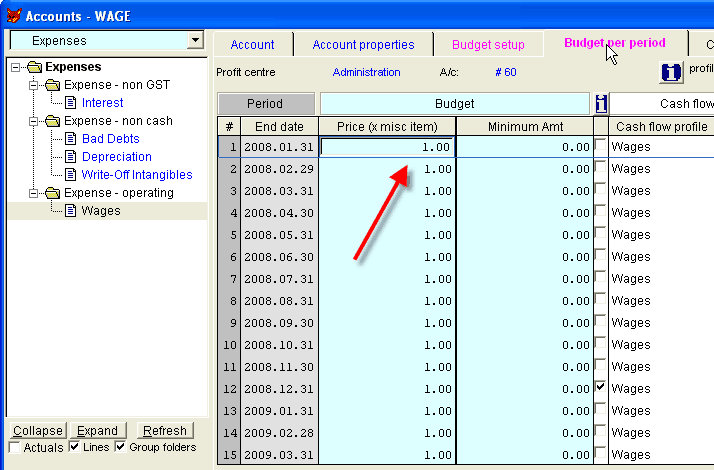Enter a 1.00 for the price for every period.
The profit and loss for wages will now be calculated for each period. You can see this in Results –view.How to instruct the program to divide the annual wages bill into 12 equal payments?

For budget cash flow use the technique discussed in the Help file for Tutorial 7. ‘Irregular expense payments’. Untick all the tick marks except for the 12th period.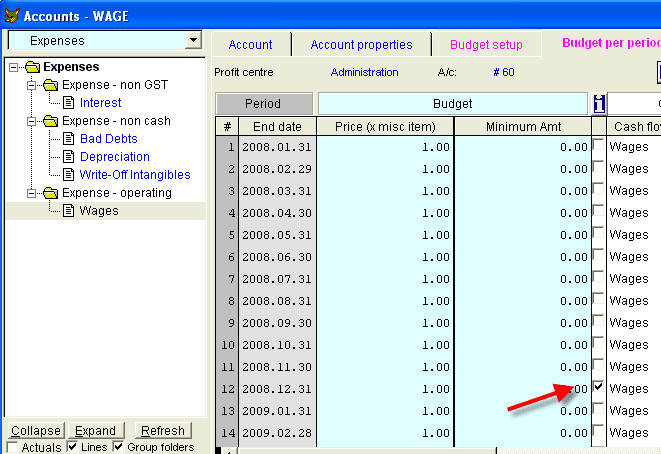The software will not process the monthly cash flows because they are unticked- instead it will calculate the total at the end of the 12th period (because it is ticked).

So all that remains is to create a cash flow profile to allocate the total to each month. As there are 12 periods, the amount to pay each month is:   100%/12 = 8.33

New cash flow profile for wages

Notice that a new cash flow profile has been created called Wages. Right-click it and choose View profile.

The profile has been split over 12 periods.The allocation is spread evenly over the 12 periods. Note that there will be some minor rounding differences, as to get the total to add up to 100% we changed some rows to 8.34 instead of 8.33.

There are 12 rows, allocated to period 0 (current period), -1, -2 … to -11.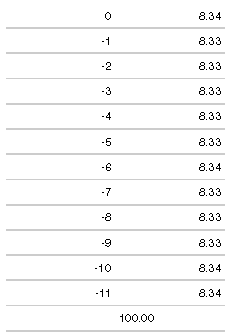Although the Wages profile has been copied to all periods in the budget per period page, it will only be used by any row that is ticked. In our case, the 12th period is ticked. That will have the total wages bill for the year, and that will be allocated approximately evenly over each period.

You will see the results in the cash flow under Results – view.

View and report budget cash flow results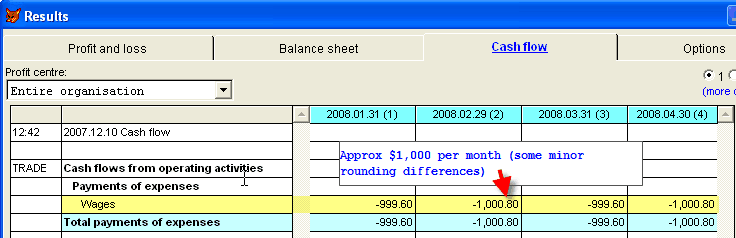When the cash flow report is printed under main report, it is usally printed without decimals and it will look like this: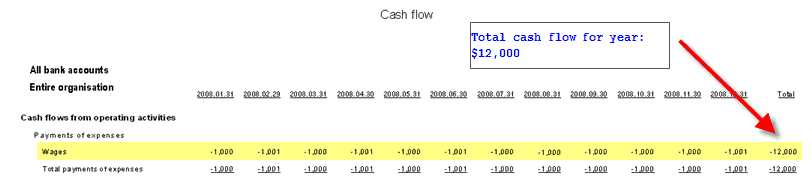Options are to use hours instead of days for the Miscellaneous item. The equation would then be changed appropriately. Also, on the budget per period page for wages, you could enter the hours per period instead of 1. Then the miscellaneous item could be Total number of hours in year, or total number of employees.